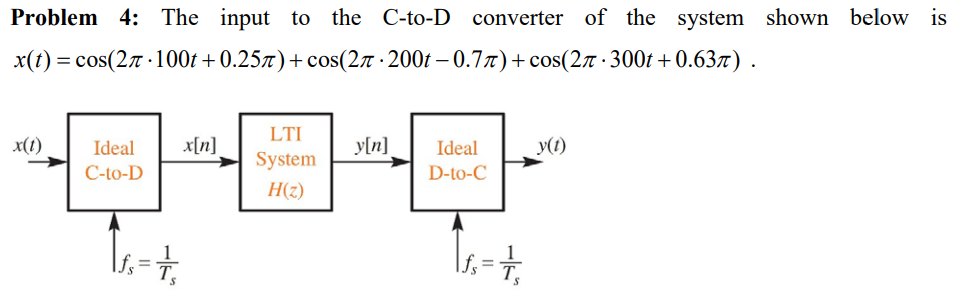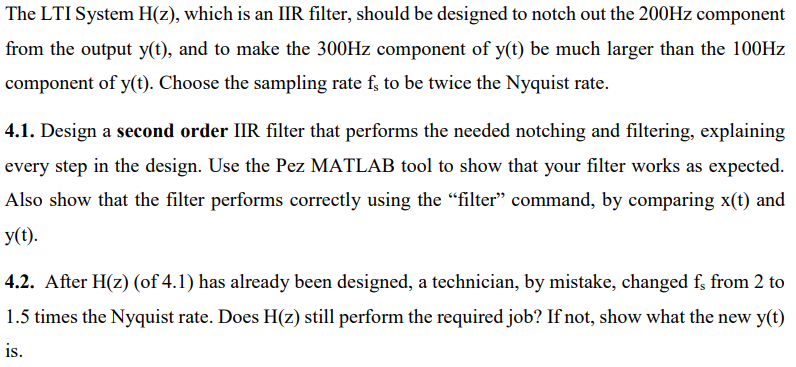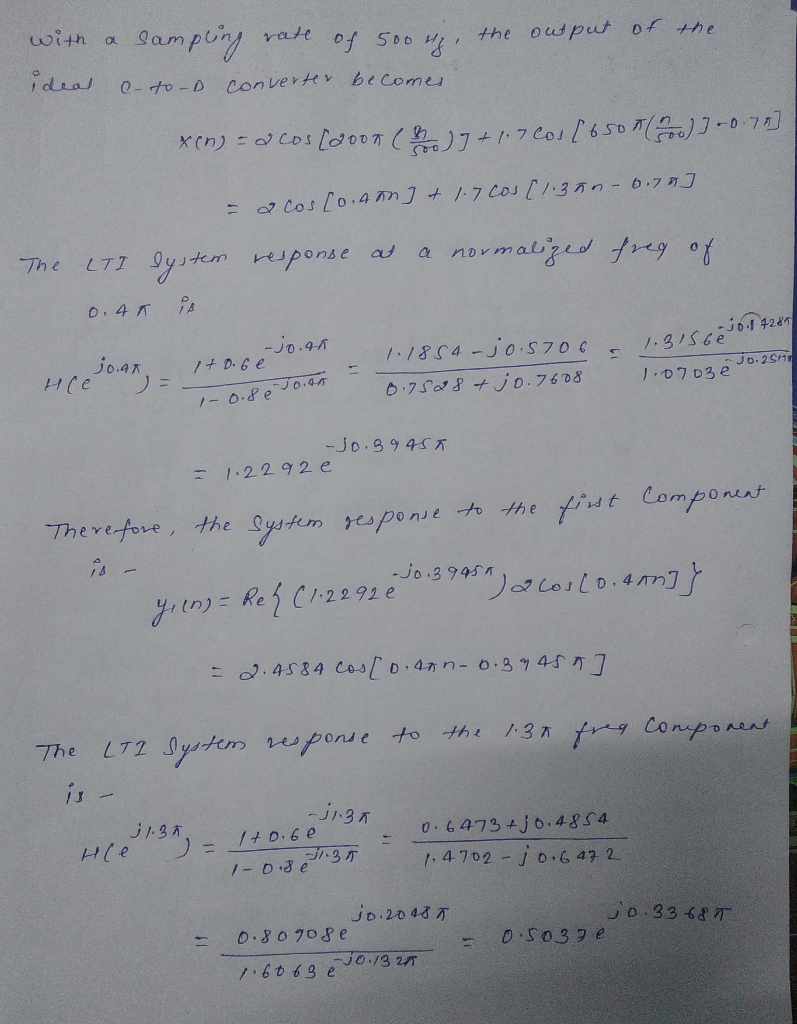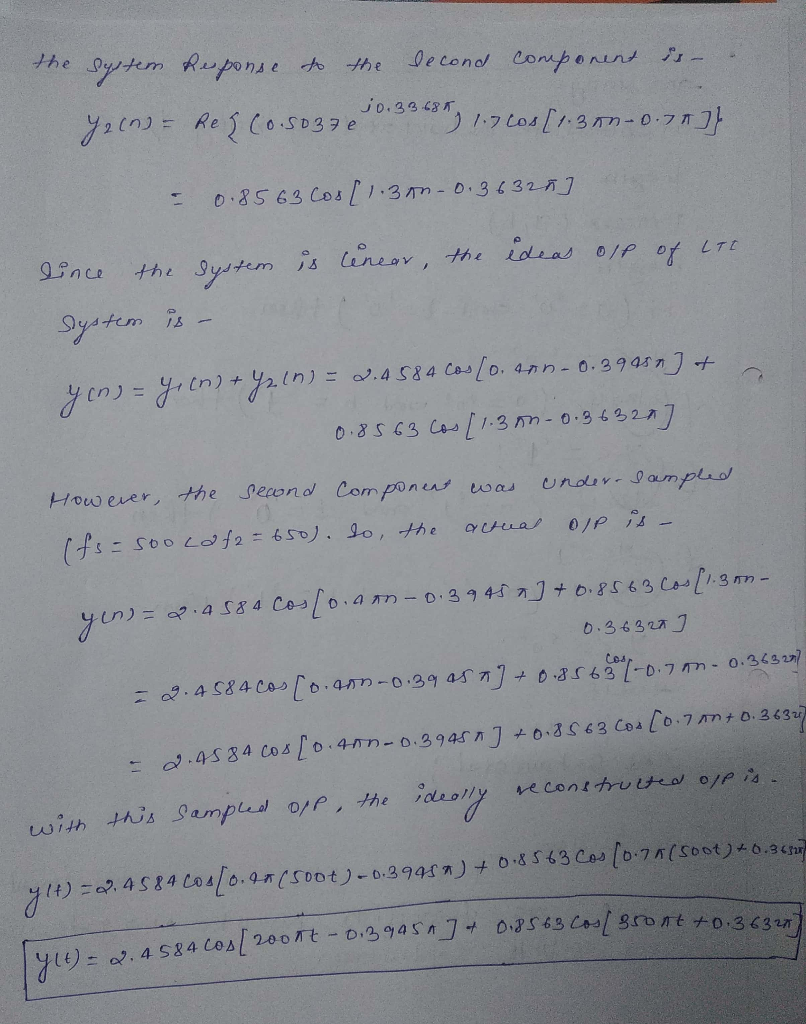# (Solved) : Question Theoretical Solution Provided Need Matlab Code Used Verify Solutions Already Foun Q42785667 . . .

The question and itstheoretical solution areprovided, I need the MATLAB code used, to verify the solutions thatare already found in thetheoreticalsolution.

*

**

*

*

Solution to the problem above is shown herebelow:

*

*

*Problem 4: The input to the C-to-D converter of the system shown below is x(t) = cos(21 · 100t +0.251)+cos(21 · 200t – 0.71)+cos(27 • 300t+0.631). x(1) x[n] ynl yi) Ideal C-to-D LTI System HZ) Ideal D-to-C The LTI System H(z), which is an IIR filter, should be designed to notch out the 200Hz component from the output y(t), and to make the 300Hz component of y(t) be much larger than the 100Hz component of y(t). Choose the sampling rate fs to be twice the Nyquist rate. 4.1. Design a second order IIR filter that performs the needed notching and filtering, explaining every step in the design. Use the Pez MATLAB tool to show that your filter works as expected. Also show that the filter performs correctly using the “filter” command, by comparing x(t) and yt). 4.2. After H(z) (of 4.1) has already been designed, a technician, by mistake, changed f, from 2 to 1.5 times the Nyquist rate. Does Hz) still perform the required job? If not, show what the new y(t) is. of the with a sampling rate of 500g, the output ideal e-to-D converter becomes X(0) = 2 cos[2007 (8) 7+ 1.7 Cos(6507(700)] -0.70] – 2 Cos (0.450] + 1.7 Cos (1.35.-6.74] The LTI System response at a normalized free of 0.4K is jo.AR ) = = À 10. 2017 -jo.4k Ito.be -0.8 é o 1-1854-jo.5706 – 1.315be 0.7528+ jo.7608 Hle 1.0703 1-O.se TOR -jo.8945 = 1.2292e Therefore, the system response to the first component 1.2292 3.995″) a cou (0.4m]} = 0.4584 Cos (0.47-0.344517 The LT 2 System response to the 1.37 frea component ! – 11:35 11.32 0.6473 +1 0.4854 1.4702 – j 0.6472 14o.be 11. 35 1-oisen Hle jo. 2018 0.80908e 10.33685 0.5037 e – – 1.6063 e 10.1325 the system Response to the becond component is- 72 (0) – Rez (0.5037 e 0.33 685 1.720s (1.350-0.70]} = 0.85 63 200[1.30-0.36322] is cinear, the ideas olf of LTE lince the system system is – yin) = (n) + y21n) = 2.4584 Cos (0,08 -0.39951) + 0.85 63 cos (1. 3m – 0.36320] However, the second component was under-sampled (fs=soo Lafa = 650). So, the actua oll is- yen=2.4584 Cos[0.0 -0.3945 a ] +0.8563 Cos (1.350- 0. 36320] – 2.4 584 cos (o.aon-0.39 as a] +0.85 680 10.00 – 0.363277 2.4584 cos [0.400 -039451] +0.8563 Cou(0.70+ 0.36327 this sampled opp, the ideally reconstructed off is. with 44) = 24584 Cos/0.97(500+)-0.39484) +08563 Cos (0.70(500+)*0.36 coacos 2oont -0. 39 957 of 0.85 63 Cos/8ront +0.3622 – 2.4584 cos/2oont – 0.2gas. Show transcribed image text Problem 4: The input to the C-to-D converter of the system shown below is x(t) = cos(21 · 100t +0.251)+cos(21 · 200t – 0.71)+cos(27 • 300t+0.631). x(1) x[n] ynl yi) Ideal C-to-D LTI System HZ) Ideal D-to-C
The LTI System H(z), which is an IIR filter, should be designed to notch out the 200Hz component from the output y(t), and to make the 300Hz component of y(t) be much larger than the 100Hz component of y(t). Choose the sampling rate fs to be twice the Nyquist rate. 4.1. Design a second order IIR filter that performs the needed notching and filtering, explaining every step in the design. Use the Pez MATLAB tool to show that your filter works as expected. Also show that the filter performs correctly using the “filter” command, by comparing x(t) and yt). 4.2. After H(z) (of 4.1) has already been designed, a technician, by mistake, changed f, from 2 to 1.5 times the Nyquist rate. Does Hz) still perform the required job? If not, show what the new y(t) is.
of the with a sampling rate of 500g, the output ideal e-to-D converter becomes X(0) = 2 cos[2007 (8) 7+ 1.7 Cos(6507(700)] -0.70] – 2 Cos (0.450] + 1.7 Cos (1.35.-6.74] The LTI System response at a normalized free of 0.4K is jo.AR ) = = À 10. 2017 -jo.4k Ito.be -0.8 é o 1-1854-jo.5706 – 1.315be 0.7528+ jo.7608 Hle 1.0703 1-O.se TOR -jo.8945 = 1.2292e Therefore, the system response to the first component 1.2292 3.995″) a cou (0.4m]} = 0.4584 Cos (0.47-0.344517 The LT 2 System response to the 1.37 frea component ! – 11:35 11.32 0.6473 +1 0.4854 1.4702 – j 0.6472 14o.be 11. 35 1-oisen Hle jo. 2018 0.80908e 10.33685 0.5037 e – – 1.6063 e 10.1325
the system Response to the becond component is- 72 (0) – Rez (0.5037 e 0.33 685 1.720s (1.350-0.70]} = 0.85 63 200[1.30-0.36322] is cinear, the ideas olf of LTE lince the system system is – yin) = (n) + y21n) = 2.4584 Cos (0,08 -0.39951) + 0.85 63 cos (1. 3m – 0.36320] However, the second component was under-sampled (fs=soo Lafa = 650). So, the actua oll is- yen=2.4584 Cos[0.0 -0.3945 a ] +0.8563 Cos (1.350- 0. 36320] – 2.4 584 cos (o.aon-0.39 as a] +0.85 680 10.00 – 0.363277 2.4584 cos [0.400 -039451] +0.8563 Cou(0.70+ 0.36327 this sampled opp, the ideally reconstructed off is. with 44) = 24584 Cos/0.97(500+)-0.39484) +08563 Cos (0.70(500+)*0.36 coacos 2oont -0. 39 957 of 0.85 63 Cos/8ront +0.3622 – 2.4584 cos/2oont – 0.2gas.

Answer to The question and its theoretical solution are provided, I need the MATLAB code used, to verify the solutions that are al…

We are the best freelance writing portal. Looking for online writing, editing or proofreading jobs? We have plenty of writing assignments to handle.